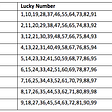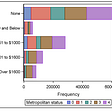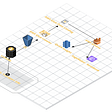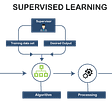# A Support Vector Machine (SVM) with Kernels tutorial using Banking data

Support Vector Machines are a branch in Machine Learning which has several applications in solving real world problems. In an example where there is a dataset and you are expected to do Binary Classification, it is very much like plotting a graph and drawing a single line to divide the different classes.

To start this exercise, we will need a few tools. Install Python programming language and set your environment variables correctly in order to proceed with the next steps. Then you must install Jupyter Notebook and download this dataset which consists of banking data from a Portuguese bank.

https://archive.ics.uci.edu/ml/datasets/bank+marketing#

Now let’s proceed with the following steps before cleaning our data.

If not used before, it is essential to install scipy, pandas, sklearn,imblearn, matplotlib and seaborn using pip. These are useful libraries to be used.

Import pandas library as below and load the csv file from the storage.

We need to ensure that the data we are using is free from missing values and outliers that will make us calculate messy test results.

If we use the head() function, we can see the data below.

Sometimes there maybe instances where there are empty value cells such as null or NaN. These must be removed as below but first we need to check if there are any null values using df.isnull().any() but this dataset doesn’t have any null values.

As we have no missing values to remove, we can proceed with removing the outliers if there are any.

Outliers are very large or very small values that appear infrequently and outside a boxplot range. These out of range values may be errors and we must remove them so that we can get more accurate predictions and have less variations among the available features.

To get a basic idea of the outliers, we use the boxplot() function. This must be used in each they are not very clear but we can visualize individual boxplots. Below is an example for one boxplot (campaign column).

So it is understandable that these can be calculation errors and therefore we must remove them as the following using a row by deletion.

Now we will get a boxplot as seen above. There are still outliers but we only delete the ones that are significant and not continuous as seen above. In fact it is the only boxplot that will show a significant outlier since it has been specified that duration column should be dropped for accurate predictions.

Now we have a ‘cleaner’ dataset. Let’s move onto the standardization.

Here we look at plotting histograms and QQ plots in order to understand the distribution of our data. Then we apply transformation codes to change the distribution of the data if it is right skewed or left skewed. Skewness results in long tails where there is fewer data in that range. This will cause errors in predictions because there are not enough values in the tail range.

As seen above, there are several histograms that have significant gaps in the distribution but are also skewed. We can easily transform age and campaign which is right skewed. We need to import scipy and matplotlib libraries for this.

The age column is right skewed. For this we use a square root transformation.

The campaign column is right skewed. For this we use a logarithmic transformation by using square root.

`import numpy as npfrom sklearn.preprocessing import FunctionTransformerdata = df.copy()# create the function transformer object with logarithm transformationlog_transformer = FunctionTransformer(np.sqrt, validate=True) #Right skewsquare_transformer = FunctionTransformer(np.square, validate=True) #left skew# apply the transformation to your datadata_age = log_transformer.transform(data[[‘age’]])data_previous = log_transformer.transform(data[[‘previous’]])data_campaign = log_transformer.transform(data[[‘campaign’]])data_nr = square_transformer.transform(data[[‘nr_employed’]])data[‘age’]=data_agedata[‘previous’]=data_previousdata[‘campaign’]=data_campaigndata[‘nr_employed’]=data_nr`

We import numpy and FunctionTransformer. By creating two transformations, square root transformation for right skew and square transformation for left skew, we plus in the relevant columns to be transformed and assign it back to the data dataframe. If you run hist() again, you will see a result as below.

We can see a small change in the shape because of the standardization. Next we move onto scaling the numerical columns.

Scaling is also known as z score normalisation. We are normalising the values to the standard deviation so that it will provide more accurate results in the later steps.

`#feature scalingfrom sklearn.preprocessing import StandardScalernumerical_df = data.select_dtypes(include=np.number)categorical_df = data.select_dtypes(exclude=np.number)# create the scaler objectscaler = StandardScaler()data_scaled = pd.DataFrame(numerical_df, columns=numerical_df.columns)# fit the scaler to the datascaler.fit(data_scaled)train_scaled = scaler.transform(data_scaled)df_scale = pd.DataFrame(train_scaled, columns=numerical_df.columns) #include column so name appearsdf_scale.describe()`

We import StandardScaler to perform scaling. We will only select columns that have continuous data. Once we fit the new data into the scaler model and convert it to a dataframe, we end up with values as below.

After standardizing the numerical data, we need to concatenate the categorical data and drop the null value row, otherwise this will cause an error in the step where we perform Principal Component Analysis.

`df_scaled = pd.concat([df_scale,categorical_df], axis = 1)df_scaled=df_scaled.dropna(axis=0)df_scaled.head()`

The categorical columns need to be coded in a way so that they can be used for principal component analysis. String values cannot be used.

`#feature coding before SEABORN matrixdf_scaled[‘job’] = df_scaled[‘job’].astype(‘category’).cat.codesdf_scaled[‘marital’].astype(‘category’).cat.codesdf_scaled[‘education’].astype(‘category’).cat.codesdf_scaled[‘default’].astype(‘category’).cat.codesdf_scaled[‘housing’].astype(‘category’).cat.codesdf_scaled[‘loan’] = df_scaled[‘loan’].astype(‘category’).cat.codesdf_scaled[‘contact’].astype(‘category’).cat.codesdf_scaled[‘month’] = df_scaled[‘month’].astype(‘category’).cat.codesdf_scaled[‘day_of_week’].astype(‘category’).cat.codesdf_scaled[‘pdays’] = df_scaled[‘pdays’].astype(‘category’).cat.codesdf_scaled[‘poutcome’].astype(‘category’).cat.codesdf_scaled[‘y’] = df_scaled[‘y’].astype(‘category’).cat.codes`

We change the datatype to int for all the columns by using cat.codes and now we move onto the Correlation Matrix.

Correlations are how well variables affect the behaviour of other variables. This gives us some understanding as to which variables are dependent and independent.

`#seaborn heatmaps for correlationimport seaborn as snsdf_corr = df_scaled.copy()#removing features that are not highly correlated with target but with other variablesdf_corr = df_corr.drop(columns[‘emp_var_rate’,’euribor3m’,’cons_price_idx’])corr_mat = df_corr.corr()plt.figure(figsize=(16,10))sns.heatmap(corr_mat, annot = True)plt.title(“Correlation matrix of Banking data”)plt.xlabel(“Banking features”)plt.ylabel(“Banking features”)plt.show()`

We need to import the seaborn library. We copy the scaled dataframe and we apply the above functions to create the correlation matrix table. I have removed several features that have a very high correlation to each other but don’t have a high correlation with our target variable. These must be removed to give a fair prediction.

This colour coded heatmap you see above indicates the correlations of each variables. Theoretically speaking, you need values that are close to +1 or -1 in order to consider it as a strong correlation. We can also see values that close to -0.5 but we can ignore these features as it is not a very strong correlation.

Now we do the Principal Component Analysis since we have some idea about the variables that are dependent and independent.

`#Principle component analysisfrom sklearn.decomposition import PCAnumerical_df = df_corr.select_dtypes(include=np.number)categorical_df = df_corr.select_dtypes(exclude=np.number)features = numerical_df.columns X = df_corr.loc[:, features].valuesY = df_corr.loc[:,[‘y’]].valuesX = StandardScaler().fit_transform(X)pca = PCA(n_components=15) #n_components=5X_pca = pca.fit_transform(X) principalDf = pd.DataFrame(data = X_pca)principalDf.head(10)`

We import PCA and we list the features to be reduced in PCA and we exclude y column because it is the target variable.

As seen above, we get 15 columns shown in the explained ratio variance, we get an array and the total is approximately 95%, which means that we have extracted the significant features that have the most variance to the machine learning model. These are the features that will give us significant results.

`finalDf = pd.concat([principalDf, df_scaled[[‘y’]]], axis = 1)finalDf=finalDf.dropna()`

We need to add back the y column so we concatenate the dataframe components and get a result as above. We have almost reached the last step which is building the machine learning model. Again, we use the dropna() function to remove NaN values (otherwise the model won’t work).

Class Imbalance is when we have a very significant difference in the distribution of the target variable. These heavily affects prediction models because one output will be significantly low and will be sampled very unevenly. Therefore we must try to replicate rows in order to balance the yes and no output.

`#Removing classs imbalancefrom imblearn.over_sampling import SMOTEfrom sklearn.model_selection import train_test_splity_true = finalDf[‘y’]X = finalDf.drop(‘y’, axis=1)os = SMOTE(random_state=0)X_class_train, X_test, y_class_train, y_test = train_test_split(X, y_true, test_size=0.2, random_state=0)columns = X_class_train.columnsdata_X, data_y = os.fit_resample(X_class_train, y_class_train)smoted_X = pd.DataFrame(data=data_X,columns=columns )smoted_y= pd.DataFrame(data=data_y,columns=[‘y’])`

We then assign variables to train and test each for X and Y. We use a 80 to 20 ratio to split the training and test data. We should install imblearn and import SMOTE to resample our dataset.

This is the output we get that shows the balanced output classes of the training dataset. We do not do this for the testing dataset because we had enough training to the 80% of the training dataset and would try to give a better accuracy as compared to not using SMOTE.

This is where the predictions happen. We must import the relevant sklearn libraries in order to use svm. In this example we can see that the SVC which is SVM with Kernels having different parameters called kernel, which is the kernel type and C, gamma variables that are to be changed according to the dataset.

By using and rbf kernel and setting C=1 and gamma=0.5, we get an accuracy of 92% for 0 labels and 39% for 1 labels. Below is a confusion matrix which shows how the category labels are predicted.

We need to keep in mind that results can vary depending on the test split. By trying different combinations, it was found that having a linear kernel regardless of the C and Gamma values will give a 54% accuracy for the 1 labels but the model takes a very long time to train. For rbf, using C=100 and Gamma =0.1 gives a good prediction result of 39% accuracy, 46% recall and 40% f-score. This is better than the result shown but these values differ from the dataset split. Usually the best accuracy level is between 50–60%.

Using Python and Jupyter Notebook, assisted by many useful libraries, we were able to use Support Vector Machine with Kernels to predict the target variable, if the customer is going to subscribe to the bank offer or not. Test results were average but given the imbalance of data, we can conclude that the prediction model did a fair job in predicting the output.

The Python code that was used to do all the steps is given below.

--

--

--

Data Science enthusiast, journalist and public speaker. IT Undergraduate at University of Moratuwa

Love podcasts or audiobooks? Learn on the go with our new app.

## Numerology & Your Lucky Lottery Number## SAS statistical analysis programming## Central Limit Theorem — Statistics## Food Industry and Technology## GTFS RealTime data collection, storage and processing with AWS services## Why industrial companies should hire data scientists## A General Guide to Data Visualization with Python for Predictive Modelling## Akhila Seneviratne

Data Science enthusiast, journalist and public speaker. IT Undergraduate at University of Moratuwa

## How Are Variables’ Splitting Value Decided in a Decision Tree?## Machine Learning Fundamentals## Linguistic Neutrality Project — Part 1: Does linguistic neutrality exist?## Programming Language(s) to Learn Based on Your Goals